﻿ 基于复合结构的两级空化发生器设计优化及数值模拟
 舰船科学技术2020, Vol. 42Issue (2): 29-32, 37PDF

Design optimization and numerical simulation of two-stage cavitation generator based on composite structure
YANG Xing-lin, SHI Yuan-yuan, YAN Li-kun, CHENG Lin, LI Zi-qiang
School of Energy and Power, Jiangsu University of Science and Technology, Zhenjiang 212000, China
Abstract: Based on the research of the related cavitation generator device, the Fluent software is used to design the cavitation generator of the composite structure with three different layouts of orifice plate and venturi tube under the premise of certain boundary conditions. Numerical simulation is to investigate the cavitation effect of three cavitation generators and the effect of different orifice arrangements on cavitation. By comparing and analyzing the velocity cloud image, the turbulent energy cloud image, the vapor content rate cloud map and the distribution scatter plot obtained by simulation, the results show that the cavitation effect of the two-stage cavitation generator of the composite structure is significantly improved, and the different arrangement modes The orifice plate has a significant effect on the cavitation effect of the two-stage cavitation generator of the composite structure. When the orifice plate is a radial distribution of the central hole, the core zone of the cavitation generator has the highest average gas content and the most cavitation effect. Significant.
Key words: two-stage cavitation     cavitation generator     flow field analysis     numerical simulation     optimization design
0 引　言

1 数值理论计算

1.1 湍流模型

 $\frac{{\partial {{u}}}}{{\partial {{x}}}} + \frac{{\partial {{v}}}}{{\partial {{y}}}} = 0;$ (1)

 $\begin{split} &\frac{{\partial {{y}}}}{{\partial {{{x}}_{{j}}}}}{\rm{\rho }}{{\rm{\mu }}_{{i}}}{{\rm{\mu }}_{{j}}} = \\ & - \frac{{\partial {{p}}}}{{\partial {{{x}}_{m{i}}}}} + \frac{{\partial \left( {{\rm{\eta }}\partial {\rm{\mu }}{/_{{i}}}\partial {{\rm{\mu }}_{\rm{j}}} - {\rm{\rho }}\overline {{\rm{\mu }}_{{i}}^{\rm{'}}{\rm{\mu }}_{{j}}^{\rm{'}}} } \right)}}{{\partial {{\rm{x}}_{{j}}}}},\;\;\;\;{{i}},{{j}} = 1,2,3; \end{split}$ (2)

 $\begin{split} &\frac{{\partial \left( {{{\rho k}}} \right)}}{{\partial {{t}}}} + \frac{{\partial \left( {{\rm{\rho k}}{{\rm{\mu }}_{{i}}}} \right)}}{{\partial {{{x}}_{\rm{i}}}}} = \frac{\partial }{{\partial {{{x}}_{{j}}}}}\left[ {\left( {{\rm{\mu }} + \frac{{{{\rm{\mu }}_{{j}}}}}{{{{\rm{\sigma }}_{\rm{k}}}}}} \right)\frac{{\partial {{k}}}}{{\partial {{{x}}_{{j}}}}}} \right] + \\ &{{{G}}_{{k}}} + {{{G}}_{{b}}} - {\rm{\rho \varepsilon }} - {{{Y}}_{{M}}} + {{{S}}_{{k}}}; \end{split}$ (3)

 $\begin{split} &\frac{\partial }{{\partial {{t}}}}{\rm{\rho \varepsilon }} + \frac{\partial }{{\partial {{{x}}_{\rm{i}}}}}\left( {{\rm{\rho \varepsilon }}{{\rm{\mu }}_{\rm{i}}}} \right) = \frac{\partial }{{\partial {{{x}}_{\rm{j}}}}}\left[ {\left( {{\rm{\mu }} + \frac{{{{\rm{\mu }}_{{j}}}}}{{{{\rm{\sigma }}_{{k}}}}}} \right)\frac{{\partial {\rm{\varepsilon }}}}{{\partial {{{x}}_{{j}}}}}} \right] + \\ &{{{C}}_{1{\rm{\varepsilon }}}}\frac{{\rm{\varepsilon }}}{{{k}}}\left( {{{{G}}_{{k}}} + {{{C}}_{3{\rm{\varepsilon }}}}{{{G}}_{{b}}}} \right) - {{{C}}_{2{\rm{\varepsilon }}}}{\rm{\rho }}\frac{{{{\rm{\varepsilon }}^2}}}{{{k}}} + {{m{S}}_{\rm{\varepsilon }}}{\text{。}} \end{split}$ (4)

1.2 空化模型

 ${\text{当}}{{p}} < {{{p}}_{\rm{v}}}\;\;{{{R}}_{{e}}} = \frac{{{{\rm{\rho }}_{\rm{v}}}{{\rm{\rho }}_{\rm{l}}}}}{{\rm{\rho }}}{\rm{\alpha }}\left( {1 - {\rm{\alpha }}} \right)\frac{3}{{{{{R}}_{\rm{B}}}}}\sqrt {\frac{2}{3}\frac{{{{{p}}_{{v}}} - {{p}}}}{{{{\rm{\rho }}_{\rm{l}}}}}},$ (5)
 ${\text{当}}{{p}} < {{{p}}_{\rm{v}}}\;\;{{{R}}_{{c}}} = \frac{{{{\rm{\rho }}_{\rm{v}}}{{\rm{\rho }}_{\rm{l}}}}}{{\rm{\rho }}}{\rm{\alpha }}\left( {1 - {\rm{\alpha }}} \right)\frac{3}{{{{{R}}_{{B}}}}}\sqrt {\frac{2}{3}\frac{{{{p}} - {{{p}}_{\rm{v}}}}}{{{{\rm{\rho }}_{\rm{l}}}}}}{\text{。}}$ (6)
2 计算模型及参数 2.1 数学模型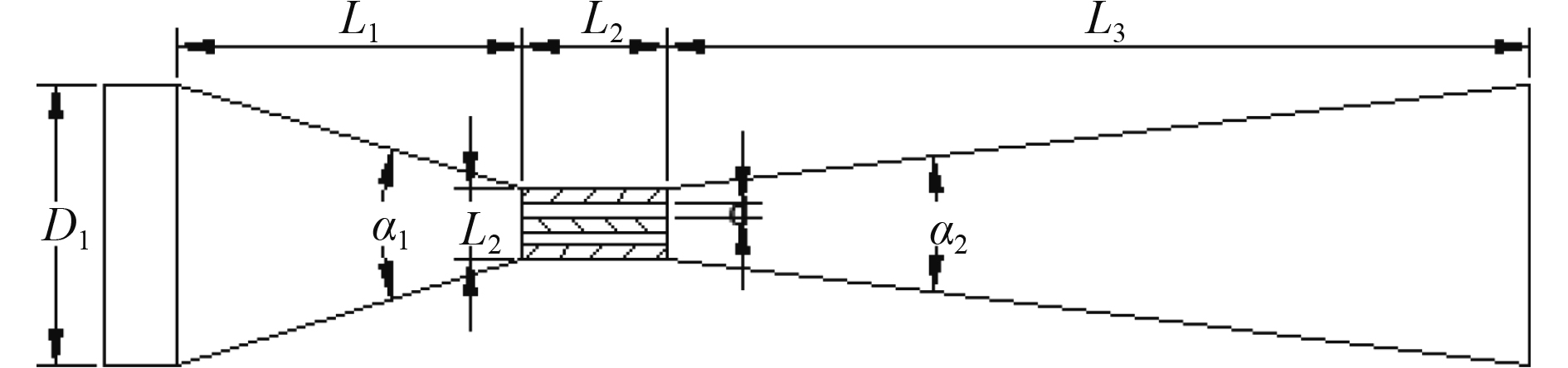图 1 复合结构空化发生器结构示意图 Fig. 1 Schematic diagram of composite structure cavitation generator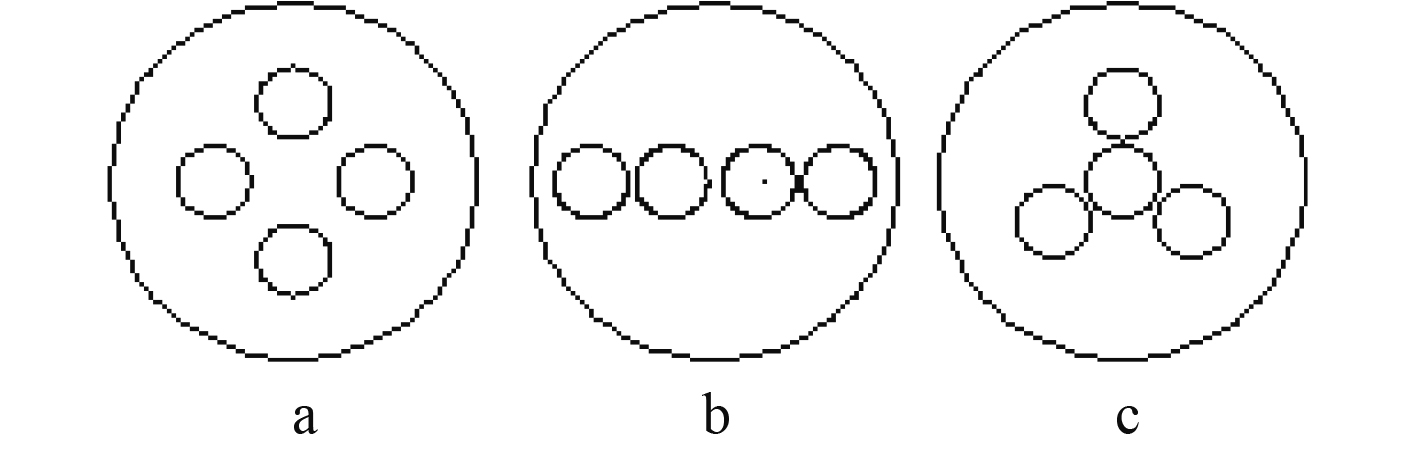图 2 孔板孔的排布方式 Fig. 2 Arrangement of orifice holes
2.2 网格划分及边界条件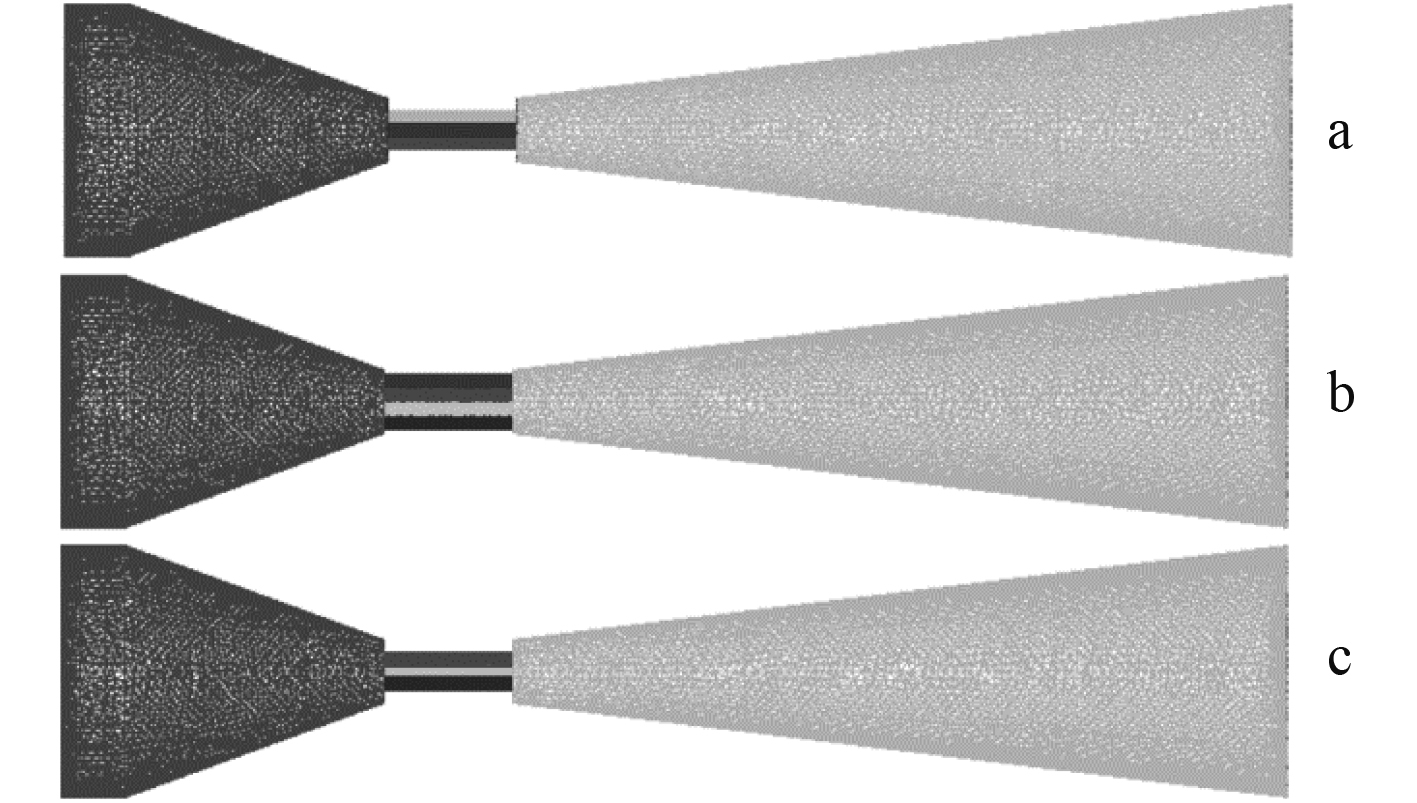图 3 空化发生器的网格示意图 Fig. 3 Schematic diagram of the cavitation generators
3 计算结果及分析

3.1 速度分布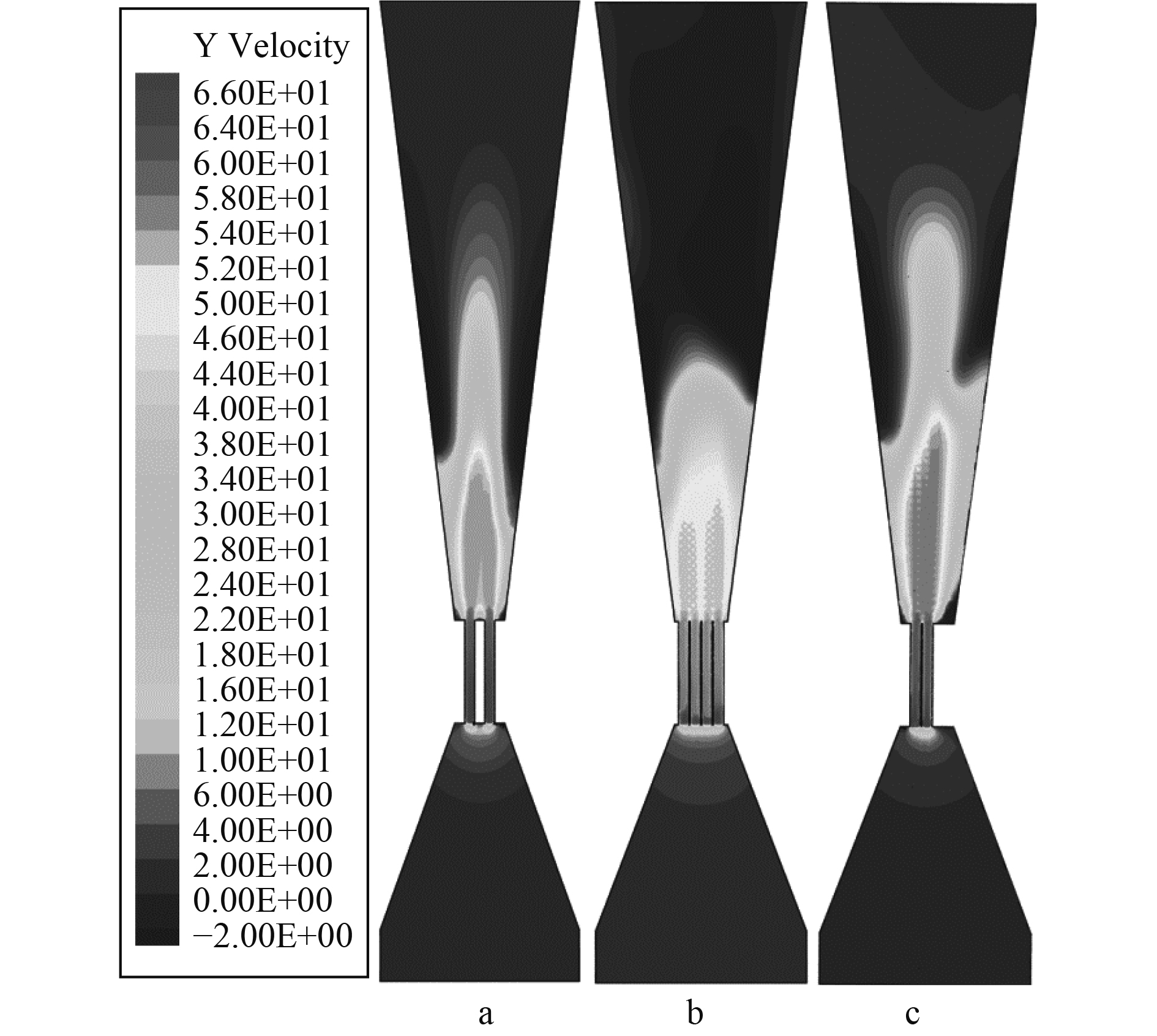图 4 空化发生器的速度云图 Fig. 4 Cavitation generator speed cloud
3.2 湍动能分布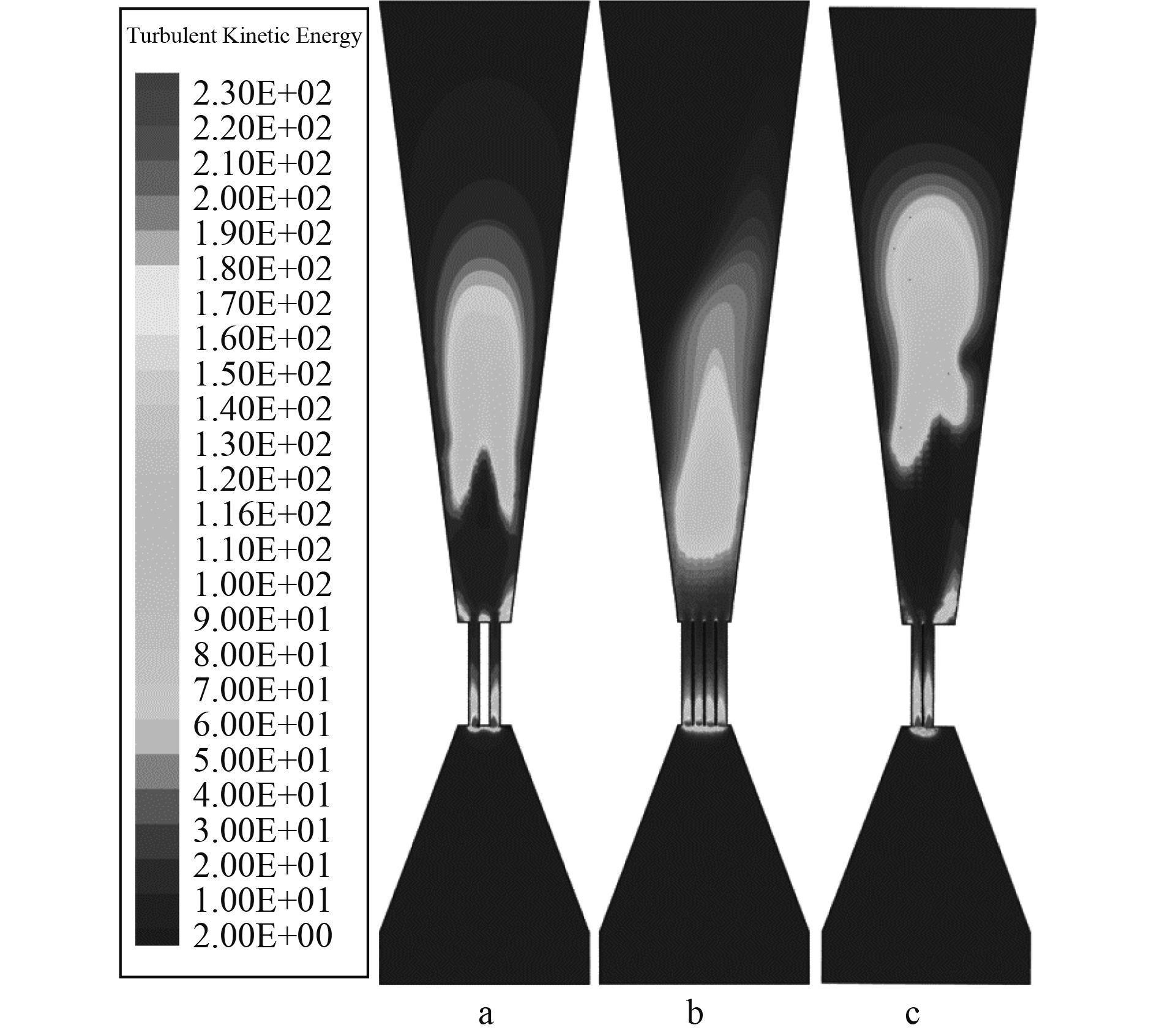图 5 空化发生器的湍动能云图 Fig. 5 The kinetic energy cloud diagram of the cavitation generator
3.3 汽含率分布云图和散点图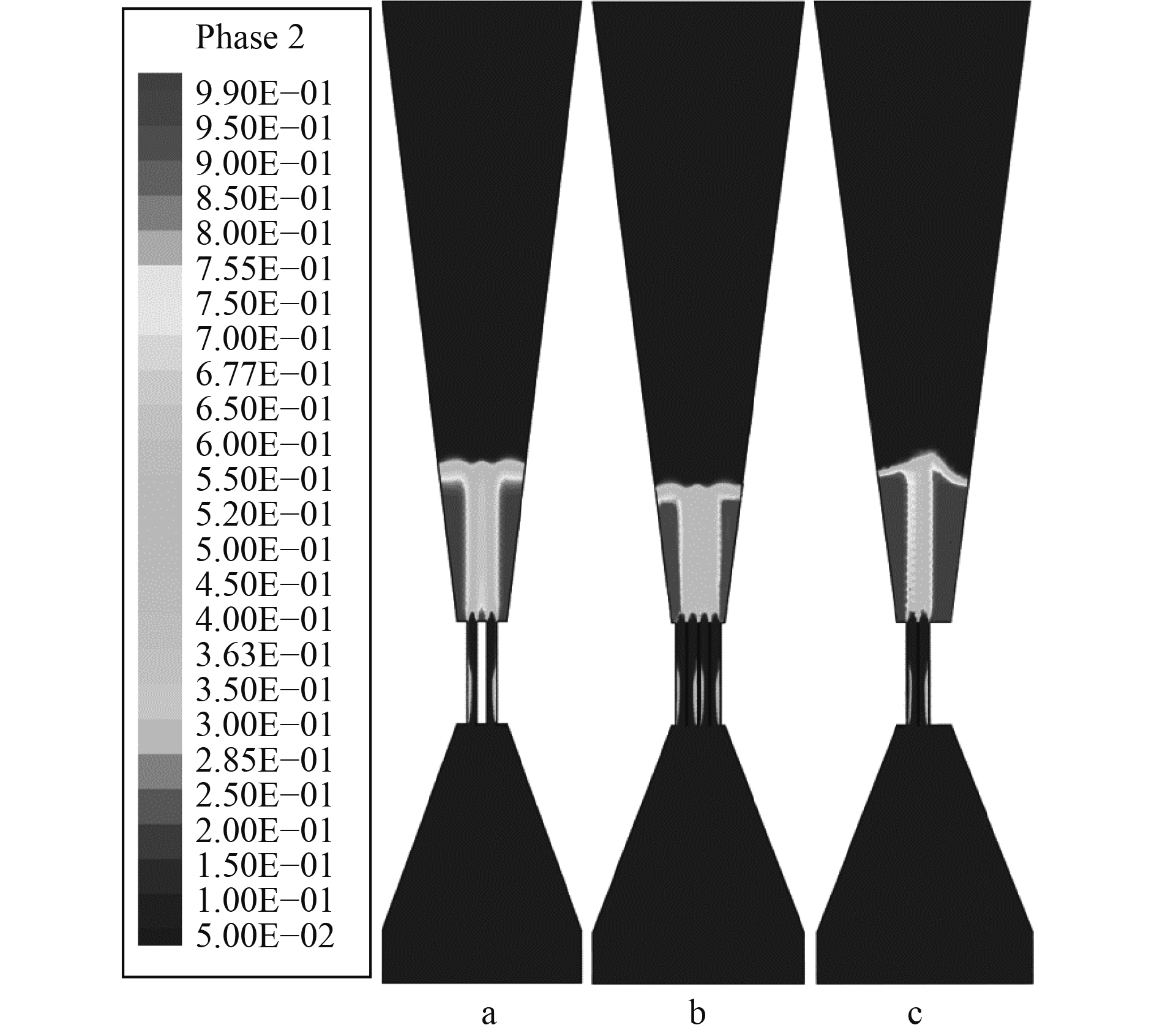图 6 空化发生器的汽含率云图 Fig. 6 Cavitation rate of the cavitation generator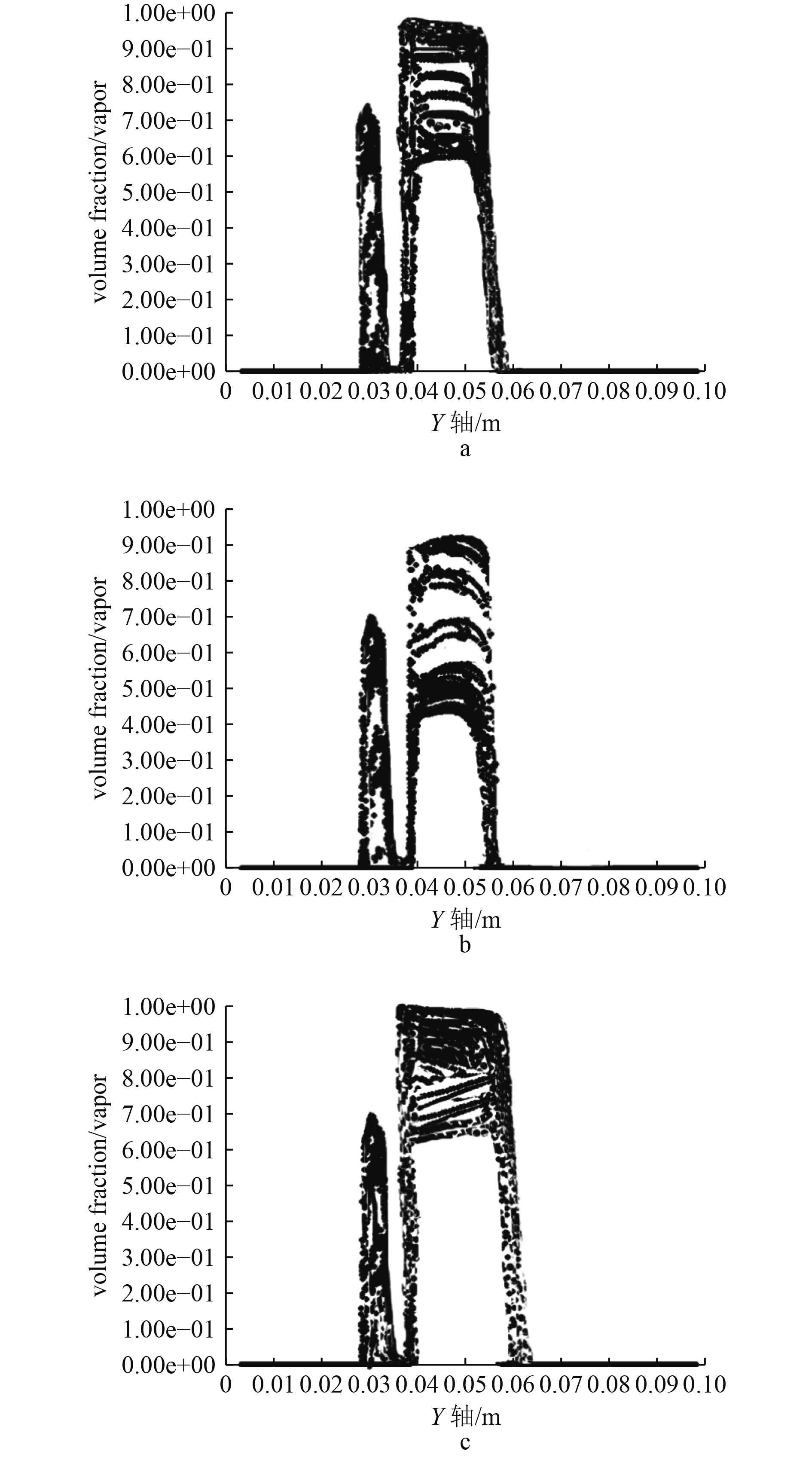图 7 空化发生器汽含率分布散点图 Fig. 7 Scatter plot of vapor content distribution of cavitation generator
4 结　语

1）本文中所设计的孔板孔道嵌套在文丘里管喉部组成的复合结构两级空化发生器可以发生2次空化，最高汽含率可以达到99%。该装置经历2次速度、湍动能的变化，可以产生更多的空化气泡，一定程度上大大提高了空化发生器的整体空化效果。

2）不同的孔板孔道排布方式影响了空化发生器内流场分布情况和大小，对速度场和湍动能产生的位置和大小范围也有影响，同时空化发生器核心区的平均汽含率也有影响。

3）本文对比分析了3种不同的两级复合结构空化发生器，在相同边界条件下，带中心孔的辐射状排布的孔板与文丘里管喉部完全嵌套所组成的复合结构空化发生器速度场和湍动能分布范围最大，汽含率最高可达99%，空化发生器范围也最大，综合对比可知，此空化发生器装置的空化性能最好。

  HAN B, JU D Y. Compressive residual stress induced by water cavitation peening: A finite element analysis[J]. Material and Design, 2009, 30(8): 3325-3332. DOI:10.1016/j.matdes.2008.11.029  管金发, 邓松圣, 张攀峰. 文丘里管空化装置设计与数值模拟[J]. 后勤工程学院学报, 2012, 28(3): 30-34. DOI:10.3969/j.issn.1672-7843.2012.03.007  启军, 邱家兴, 程玉胜, 等. 船舶螺旋桨空化噪声非均匀调制特性及其应用[J]. 舰船科学技术, 2017, 39(6): 18-22. DOI:10.3404/j.issn.1672-7619.2017.06.004  王超, 林杨, 胡志强, 等. 空化模型中的相变系数对不同头型的适用性研究[J]. 舰船科学技术, 2018, 40(2): 39-45. DOI:10.3404/j.issn.1672-7649.2018.02.007  王永广, 赵连玉, 邓橙, 等. 基于孔板和文丘里管复合结构空化器的空化效果数值模拟[J]. 环境工程, 2012, 30: 458-460.  王杰阳. 多孔板水力空化发生器空化过程的数值模拟研究[D]. 天津: 天津科技大学, 2015.  章昱, 李育敏, 计建炳. 孔板水力空化装置的数值模拟[J]. 化学反应工程与工艺, 2011, 27(3): 219-223. DOI:10.3969/j.issn.1001-7631.2011.03.005  张建峰, 卢晓江, 余冬梅. 孔板为发生器的水力空化装置的实验研究[J]. 轻工机械, 2008, 26(2): 15-18. DOI:10.3969/j.issn.1005-2895.2008.02.005  曾章美, 刘小兵, 兰巍, 等. 孔板和文丘里管复合结构空化器的优化设计[J]. 西华大学学报(自然科学版), 2015, 34(6): 92-95. DOI:10.3969/j.issn.1673-159X.2015.06.019